Carrasco, J. C., Oporto, G. S., Zondlo, J., and Wang, J. (2014). "Observed kinetic parameters during the torrefaction of red oak (Quercus rubra) in a pilot rotary kiln reactor," BioRes. 9(3), 5417-5437.

#### Abstract

The torrefaction of red oak (Quercus rubra) was performed in a pilot rotary kiln reactor, and the apparent kinetic results were compared with the results of torrefaction performed in a bench-scale fluidized reactor. Mass loss, gross calorific analyses, ultimate analyses, and proximate analyses were applied to the final torrefied material. The experimental torrefaction temperatures were 250, 275, 300, and 325 °C, and the experimental total torrefaction times were 20, 35, 50, and 80 min. A significant variation of the energy content occurred in the range of temperature between 275 and 300 °C, with the energy yield changing from 97.5% to 83.6%, respectively. The molar ratios H:C:O for the torrefied red oak presented a behavior independent of the experimental equipment when the temperature ranged between 250 and 325 °C. For the torrefaction process of red oak in the pilot rotary kiln reactor, a first-order reaction and one-step kinetic model were fitted with a maximum error of about 7.5% at 325 °C. The observed reaction rate constant (k) for the rotary reactor was 0.072 min-1 at 300 °C, which was 71% lower than the reaction rate constant for torrefied red oak in a bench-scale fluidized reactor. Arrhenius analysis determined an activation energy of 20.4 kJ/mol and a frequency factor of 5.22 min-1. The results suggest significant external heat and mass-transfer resistances in the rotary system.

#### Full Article

Observed Kinetic Parameters during the Torrefaction of Red Oak (Quercus rubra) in a Pilot Rotary Kiln Reactor

Juan C. Carrasco,a,b Gloria S. Oporto,a,b,* John Zondlo,c and Jingxin Wang a

The torrefaction of red oak (Quercus rubra) was performed in a pilot rotary kiln reactor, and the apparent kinetic results were compared with the results of torrefaction performed in a bench-scale fluidized reactor. Mass loss, gross calorific analyses, ultimate analyses, and proximate analyses were applied to the final torrefied material. The experimental torrefaction temperatures were 250, 275, 300, and 325 °C, and the experimental total torrefaction times were 20, 35, 50, and 80 min. A significant variation of the energy content occurred in the range of temperature between 275 and 300 °C, with the energy yield changing from 97.5% to 83.6%, respectively. The molar ratios H:C:O for the torrefied red oak presented a behavior independent of the experimental equipment when the temperature ranged between 250 and 325 °C. For the torrefaction process of red oak in the pilot rotary kiln reactor, a first-order reaction and one-step kinetic model were fitted with a maximum error of about 7.5% at 325 °C. The observed reaction rate constant (k) for the rotary reactor was 0.072 min−1 at 300 °C, which was 71% lower than the reaction rate constant for torrefied red oak in a bench-scale fluidized reactor. Arrhenius analysis determined an activation energy of 20.4 kJ/mol and a frequency factor of 5.22 min−1. The results suggest significant external heat and mass-transfer resistances in the rotary system.

Keywords: Torrefaction; Red oak; Pilot rotary kiln reactor; Kinetics; Energy yield; Mass loss

Contact information: a: Division of Forestry and Natural Resources, West Virginia University, Morgantown, WV 26506; b: Unidad de Desarrollo Tecnológico (UDT) – University of Concepcion, Chile; c: Department of Chemical Engineering, West Virginia University, Morgantown, WV 26506;

* Corresponding author: gloria.oporto@mail.wvu.edu

INTRODUCTION

Woody biomass currently comprises up to 20% of the co-combustion material in coal power plants, accounting for 0.9% of total U.S. electric power generation (Levine 2011). Furthermore, 46% of all electricity generation comes from coal power plants, in which greenhouse gas (GHG) emissions are the most important environmental issue; the GHG from coal combustion for power generation accounted for 81% of emissions from electricity generation from fossil fuels (Cuellar 2012). The low level of utilization of biomass in power plants for electric generation is due to several limitations: their low energy density compared with fossil fuels, high grinding energy requirements, and variability in moisture content (Bergman et al. 2005; Bridgeman et al. 2010). These limitations decrease the efficiency and output of a power plant. Today, it is possible to improve all these disadvantages of biomass through the torrefaction process (Prins et al. 2006a; Deng et al. 2009; Zheng et al. 2012). Torrefaction is the thermochemical treatment of biomass in the absence of oxygen at temperatures ranging between 200 and 330 °C (Bergman and Kiel 2005; Prins et al. 2006a; Ciolkosz and Wallace 2011; Carrasco et al. 2013).

The decomposition of biomass during torrefaction gives off various types of volatile species, which results in a loss of mass and chemical energy to the gas phase. Moreover, biomass loses relatively more oxygen and hydrogen compared with carbon, and therefore the remaining solid material possesses not only more uniform properties such as better grindability and high hydrophobicity, but also a higher calorific value compared with the original raw material (Prins et al.2006b; Arias et al. 2008; Oliveira and Rousset 2009; Almeida et al. 2010; Kim et al. 2012). It has been suggested that torrefied material can be used as a feedstock for combustion, for pyrolysis and liquefaction, and for gasification and co-combustion with coal in power plants (Bergman et al. 2005; Prins et al.2006b; Deng et al. 2009; Kleinschmidt 2011; Li et al. 2012; Zheng et al. 2012; Tiffany 2013).

Prior studies on torrefaction have used diverse experimental systems such as thermogravimetric analyzers, ovens, and fixed bed and fluidized reactors. So far, the most conventional system has been the thermogravimetric analyzer, in which 4 to 10 mg of material can be processed (Prins et al. 2006a; Bridgeman et al. 2008; Chen and Kuo 2011; Aziz et al. 2012). Ovens have also received some attention, and a larger amount of material (40 to 70 g) can be processed in them (Almeida et al. 2010; Bridgeman et al. 2010; Fisher et al. 2012). Fixed bed reactors have been used in some experiments, and the material to be processed ranged from 10 g to 500 g (Prins et al. 2006b; Deng et al. 2009; Oliveira and Rousset 2009; Kim et al. 2012). Finally, fluidized systems have been designed to study the thermal decomposition kinetics of wood by Barooah and Long (1976), Di Blasi and Branca (2001), Peng et al. (2012), and Carrasco et al. (2013).

Around the world, there are several universities, research institutes, and companies that are studying the main torrefaction reactor concepts at the pilot scale and/or in a demonstration plant. Among these reactors are a rotary drum, a multiple hearth furnace, and screw, moving bed, and belt reactors (Alankangas 2013; Kiel 2013). There are at least seven research institutes and companies that are focusing their studies on the rotary drum technology. Previous research on biomass torrefaction using rotary kiln reactors was not found.

It is important that the parameters of the thermal decomposition kinetics are known, as they allow the determination of the time and temperature required for the torrefaction process to achieve the desired properties of the torrefied material. For the most part, findings on the kinetics for the thermal decomposition of wood have been published for the pyrolysis process (which includes torrefaction). Thurner and Mann (1981), Reina et al. (1998), Di Blasi and Branca (2001), and Ren et al. (2013) have proposed a one-step reaction process. The authors’ main comment is that it is difficult to present a kinetic model that is widely applicable, considering the variable composition of wood and the large number of unknown chemical reactions during its thermal decomposition. Samuelsson et al. (2013) presented a new methodology for modelling the kinetics of torrefaction, but promised in a future work to apply the new kinetic methodology to the torrefaction of biomass. Peng et al. (2012) showed that most of the research performed on the thermal decomposition of wood incorporates first-order kinetic models to predict the behavior of wood pyrolysis, where torrefaction may be considered the first step of the overall pyrolysis process. Carrasco et al. (2013) presented a model for the torrefaction of red oak using a one-step reaction mechanism, a summary of which is presented below: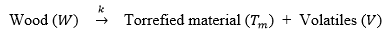The one-step reaction process can be presented as two reactions in parallel: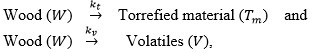where k is the overall reaction rate constant,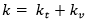, min-1kt is the reaction rate constant for the torrefied material, min-1, and kv is the reaction rate constant for the volatiles, min-1. The elementary reaction rates for the solid wood () and the torrefied material’s (Tm) decomposition over time can be presented using the following equations: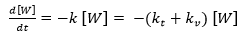(1)

and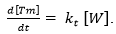(2)

The fraction of total solids (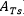) in the system after torrefaction is,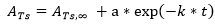, (3)

where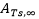is the fraction of total solids when torrefaction time is sufficiently long and a is the dimensionless kinetic parameter for a first-order reaction.

The reaction rate constant “k” can be represented by the Arrhenius equation (Eq. 4). It shows the dependence of “k” for the chemical reaction on the absolute temperature, T in K, Ea, the activation energy, “ko the frequency or pre-exponential factor, and R, the universal gas constant (8.314 J mol-1K-1).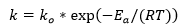(4)

This kinetic model will allow the prediction of the behavior of biomass during the torrefaction process. That is, the kinetic model will be expected to describe the changing mass yield as a function of time and temperature.

In this research a pilot rotary kiln reactor was used to determine the apparent torrefaction kinetics of a sample of red oak at temperatures between 250 and 325 °C, and the results were compared with the kinetic parameters obtained for the same red oak samples in a bench-scale fluidized reactor.

EXPERIMENTAL

Materials

Red oak was collected as wood chips (without bark) from a West Virginia sawmill. The raw material (between 0.5 and 10 cm of length) was screened to the size of 2.0 to 9.51 mm, using sieves (No.10 to 3/8in. U.S. mesh). The moisture content of the wood chips was 7% ± 1%, and they were dried until constant weight, before the torrefaction process, using an oven at 107 °C for 72 h.

Particle Size

Considering the large particle size utilized in the present study, it is necessary to determine the potential kinetic limitations during the torrefaction process. These limitations can be examined using the methodology proposed by Pyle and Zaror (1984) and Bates (2012) through the assessment of the Biot and Pyrolysis numbers, by means of the following equations,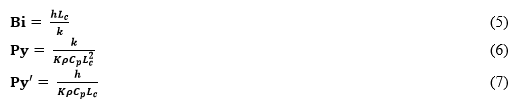where Lc is the particle characteristic length [m],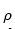is the biomass particle density [kg/m3], Cp is the biomass particle specific heat [J/kg*K], h is the external heat transfer coefficient [W/m2*K], k is the particle conductivity [W/m*K], and K is the reaction rate constant [1/s].

The Biot Number, is a dimensionless parameter, which measures the interaction between the thermal conduction inside a particle and the convection at its surface. Py, the Internal Pyrolysis number, gives a measure of the relative importance of the internal thermal conduction and the reaction rate constant. The External Pyrolysis number, Py’ gives the ratio between heat convection rate and the reaction rate. According to Pyle and Zaror (1984) the following table shows the controlling condition in the pyrolysis process.

Table 1. Controlling Conditions and Dimensionless NumbersTorrefaction Reactor

A rotary kiln reactor located inside of a furnace with automatic temperature control was used. The furnace was heated through electrical resistances. The reactor was a box made of stainless steel with the following dimensions: 600 mm long, 300 mm wide, and 300 mm high. The reactor was purged with nitrogen during operation and the volatiles were collected in a cold trap in the gas stream at the reactor exit. The furnace was equipped with a thermocouple for precise temperature control and the reactor had another thermocouple at its center to monitor the temperature inside the reactor (Fig. 1).

Approximately 827 ± 13 g of red oak raw material was used for each experiment; this amount corresponded to nearly 25% of the reactor volume. Nitrogen at a flow rate of 35 L/min was used as the inerting gas. A total of 16 samples of red oak were prepared for the torrefaction experiments; details of these experiments are presented in Table 2.

The raw material was introduced into the reactor when the system was at ambient temperature. The rotation speed of the reactor was 1 rpm. The time/temperature profiles of both the reactor and furnace were recorded simultaneously using thermocouples TIR-1 and TCR, respectively (Fig. 1).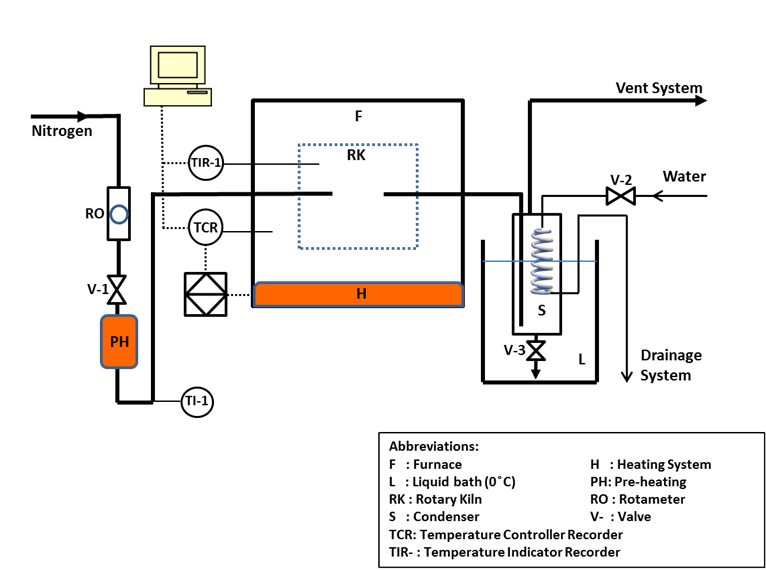Fig. 1. Pilot experimental system

Table 2. Nomenclature and Parameters of Torrefied Red Oak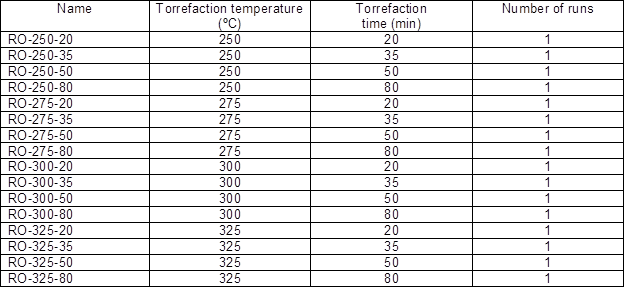In each experiment the “heat-up” time prior to the onset of torrefaction (here assumed to be 200 °C) from ambient temperature to 200 °C was approximately 25 min. The subsequent total torrefaction time is composed of two components: i) a heating time (th) of 20 min to go from 200 °C to the desired temperature and ii) a torrefaction period at the desired temperature (tt). Thus, the total torrefaction time is th + tt. For example, the experiments RO-250-20, RO-275-20, RO-300-20, and RO-325-20 considered a tt equal to 0 min and th = 20 min. By so doing, we can evaluate the mass loss provoked by th. The desired torrefaction temperature was controlled through the temperature controller/recorder (TCR). A copper condenser (S) was used to trap the condensable gases from the reactor; it used a cooling system consisting of an internal water-cooled coil (by opening V-2). The emerging non-condensable torrefaction gases were vented into the hood. When the final torrefaction time was reached, rapid quenching of the torrefied biomass was achieved through of a cooling system consisting of a flow of fresh nitrogen (by turning off the pre-heater, keeping V-1 open, and raising the nitrogen flow to 175 L/min); likewise, it was necessary to open the furnace door and circulate ambient air into the reactor with a fan.

Mass Loss

Mass loss (ML) of the samples after torrefaction was calculated using the following formula: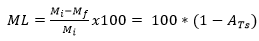, (8)

Gross Calorific Value

The gross calorific value (HCf) of the raw and torrefied red oak samples was measured using a Parr 6300 bomb calorimeter (Parr Instrument Co.; USA) following ASTM D2015 (2000). Samples between 0.5 and 1.0 grams were used for HCf determination. The equipment was calibrated using benzoic acid pellets each time a set of new determinations was initiated.

Energy Yield

The energy yields (Ey) considering different temperatures and times of torrefaction were determined using the following relationship: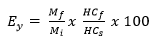(9)

where HCf is the gross calorific value of torrefied sample (kJkg−1) and HCs is the gross calorific value of raw material (kJkg−1).

Proximate Analysis

Proximate analysis of untreated and torrefied red oak was performed according to ASTM D3172-07 (2007). A proximate analyzer LECO 701 (LECO Corp.; USA) was used. Approximately two grams of material was required. The results include the determination of moisture, volatile matter, ash, and fixed carbon.

Ultimate Analysis

Ultimate analysis of untreated and torrefied red oak was performed using a ThermoQuest Elemental Analyzer, FLASH EA 1112 series (Thermo Scientific; USA), according to ASTM D3176 (1989). The amount of sample required was 1 to 2.5 mg. The elemental composition corresponds to wt% of nitrogen, carbon, sulfur, hydrogen, and oxygen (by difference). The results reported are the average of three measurements. Before and after each set of measurements a standard sample was analyzed to verify the performance of the equipment.

Kinetics

The mass loss of wood (ML) after the torrefaction experiment at a specific time and temperature was calculated according to Eq. 8. Using this mass loss, the parameter in was determined at different times and temperatures (Eq. 8). The fraction of total solids at infinite time was determined from Eq. 3.

For different experimental times (t) using known parameters ATs and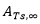, it was possible to obtain the values for “a” and “k” as functions of the torrefaction temperature through a graphic method using Eq. 3. Finally, using the natural logarithm of Eq. (3), it was possible to obtain a linear function with intercept ln(a)and slope k.

By plotting Eq. 4 with different reaction rate constants as a function of the torrefaction temperature, it was possible to determine the activation energy (Ea) and pre-exponential factor (ko).

RESULTS AND DISCUSSION

Particle Size Effects on the Kinetic Parameters

According to Bates (2012), “A kinetically controlled regime occurs for small Biot number (<1) and large Pyrolysis numbers (>10). The particle has a uniform temperature, equivalent to the reactor temperature, and the reactions occur uniformly throughout the particle at a rate roughly ten times slower than the internal or external heat transfer rate.”

The Biot Number for the torrefaction of red oak in our pilot rotary kiln reactor is equal to 0.13, and Table 3 presents the Pyrolysis Numbers for 250, 275, 300, and 325 °C. (for details see Appendix A)

Table 3. Py, and Py’ Numbers at 250, 275, 300, and 325 °CAccording to the obtained Biot and Pyrolysis Numbers, Pyle and Zaror (1984) and Bates (2012) comment that the torrefaction process presents an “external heat transfer controlled regime”. Specifically, small Biot (<1) and large Pyrolysis numbers (>1) imply that the temperature and reaction zone of the particle are uniform, but the particle temperature approaches the reactor temperature.

It is important to comment that according to Basu et al. (2013) the influence of the particle diameter (Poplar cylinder: 5 to 25 mm) on the mass and energy yield was only about 10% (using a directly heated convective reactor, torrefaction temperature: 250°C, and torrefaction time: 60 min); however, the length of the particle (from 8 to 65 mm) had a different effect, because the energy yield increased by approximately 20%, but the mass yield increased only by 10%. Likewise, Bridgeman et al. (2010) showed the influence of diameter (Miscanthus: < 4 and > 10 mm and willow: < 10 and > 20 mm) over the mass yield, had a maximum change of only 3.2% (using a horizontal tube furnace, torrefaction temperature: 230 to 290 °C, and torrefaction time: 10 to 60 min).

Therefore, considering the results presented above, it is possible to suggest that: i) the particle has an uniform temperature and the reactions occur uniformly inside of the material at a rate ten times slower than the internal heat transfer rate, ii) the controlling condition in our system is the external heat transfer rate, iii) during the torrefaction of red oak in the rotary kiln reactor, the apparent or global kinetic parameters are obtained instead of nearly intrinsic kinetic parameters as in the fluidized bed, and iv) considering that the torrefaction performed in this project is controlled mainly by external heat transfer, therefore, the scale up from the fluidized bed system to the rotary kiln system is not recommended unless further studies are performed to evaluate specifically the external resistances.

Mass Loss of Torrefied Red Oak

The change in the torrefaction temperature from 250 to 325 °C had a much more significant effect on the mass loss for the red oak than the change in residence time (from 20 to 80 min) at each temperature. For example, when the torrefaction time increased from 20 to 80 min at 250 °C, the mass loss increased from 13.3% to 19.7%, a difference of 6.4% (Table 4). In contrast, for a constant residence time of 20 min and an increase in temperature from 250 to 300 °C, the change of mass loss increased from 13.3% to 33.5%, a difference of 20.2%. These results confirm the similar findings of Bridgeman et al. (2010), Medic et al.(2012), and Carrasco et al. (2013); the torrefaction temperature is the most important parameter in terms of mass loss compared with the residence time, moisture content, and particle size.

Table 4. Mass Loss, Gross Calorific Value, and Energy Yield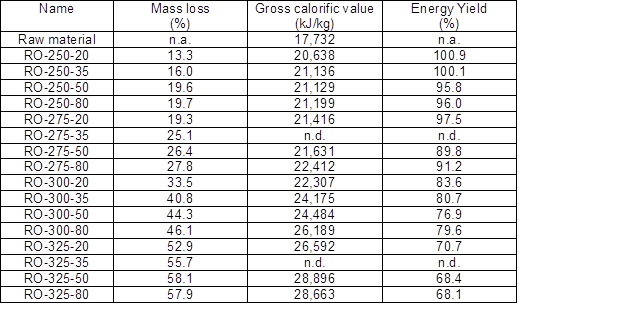n.a.: not applicable

n.d.: not determined

The differences in terms of mass loss in the rotary kiln used in this research compared with the fluidized reactor used in our previous research (Carrasco et al.2013) were assessed. Mass loss as a function of the temperature in the fluidized reactor presented higher values than those obtained in the rotary reactor. For instance, at 330 °C and 30 min of torrefaction the resulted mass loss was 75.8%; however, for the rotary kiln reactor at 325 °C and 80 min, the mass loss was 57.9%. This result suggests that when using the same raw material and similar torrefaction conditions, but different size of particles, the fluidized system presents higher reaction kinetics than the rotary kiln reactor. This is most likely caused by the poorer mixing and higher mass and energy transfer limitations in the rotary reactor.

Figure 2 shows the relationship between mass loss and torrefaction temperature when the temperature was increased from 250 to 325 °C. According to the data presented in Fig. 2, the beginning of the torrefaction temperature should be between 200 and 225 °C. Carrasco et al. (2013), Peng et al. (2012), Prins et al.(2006a), and Bergman et al. (2005) suggested that the torrefaction process should begin at about 200 °C.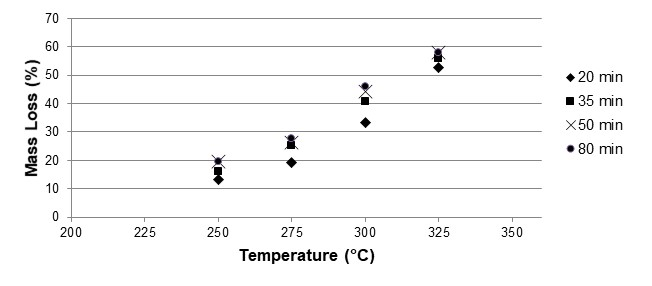Fig. 2. Mass loss of red oak at different temperatures and residence times

Gross Calorific Value

Table 4 and Fig. 3 show the gross calorific values for the raw material and for the samples after the torrefaction process.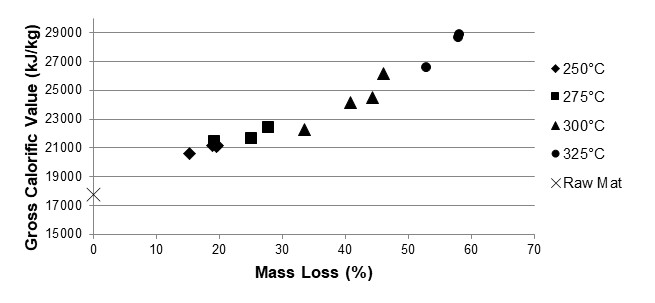Fig. 3. Gross calorific value as a function of mass loss after torrefaction

Table 4 shows that at 300 °C, the highest increment in calorific value (from 22,307 kJ/kg to 26,189 kJ/kg) was realized; this happened when the torrefaction time increased from 20 min to 80 min and the mass loss shifted from 33.5 to 46.1%. These results are consistent with those published by Aziz et al. (2012), Bates and Ghoniem (2012), Bridgeman et al. (2008), and Prins et al. (2006a), who mentioned that the more intense chemical reactions associated with the decomposition of hemicellulose, cellulose, and lignin occur between 275 and 350 °C. However, Prins et al. (2006a) recommended a temperature below 300 °C for industrial operation, because above this temperature the fast thermal cracking of cellulose may cause tar formation.

Different energy values for torrefied biomass have been found between 275 and 300 °C. Bridgeman et al. (2008) published a calorific value of 22,600 kJ/kg with a mass loss of roughly 38%; these data were obtained using a TGA at 294 °C and 45 min with wheat straw as the raw material. Almeida et al. (2010) reported a calorific value of 21,800 kJ/kg and a mass loss about of 23% for one hour at 280 °C with Eucalyptus saligna as the raw material. Carrasco et al. (2013) reported a gross calorific value of 21,200 kJ/kg using a fluidized reactor with a mass loss of roughly 40%; the raw material used was red oak, and the torrefaction process was performed at 275 °C and 10 min. In this research, Fig. 3 presents a calorific value of about 22,300 kJ/kg for a mass loss of approximately 33% at 300 °C. These results suggest that at a temperature of torrefaction between 275 and 300 °C the highest incremental change of the gross calorific value occurs in the torrefied material; however this temperature range depends on the different heating conditions, types of raw materials, various experimental devices, and the particular operating conditions.

Energy Yield

The results of the calculations for the changes in energy yield are presented in Table 4 and Fig. 4. Table 4 shows that the first two values (RO-250-20 and RO-250-35) of energy yield were greater than one hundred percent, and this represents an error in the experimental data of roughly 0.9%. At 20 min of torrefaction time, the most important reduction in the energy yield from 97.5% to 83.6% occurred between 275 and 300 °C. Kim et al. (2012) suggested that the optimal condition for torrefaction was 280 °C and 30 min, with an energy yield of 80.4%. Likewise, Almeida et al. (2010) showed that the most important decline in energy yield occurred at 280 °C and 5 h, with an energy yield of about 77.5%.

Figure 4 presents the energy yield as a function of mass loss published by different authors, such as Ohliger et al. (2013), Kim et al. (2012), Medic et al. (2012), Almeida et al. (2010), and Brigdeman et al. (2010). Ohliger et al. (2013) worked with a horizontal screw conveyor using 2,000 g of beechwood chips, with a torrefaction temperature between 270 and 300 °C. Kim et al. (2012) performed the experimental methodology using a batch reactor with 500 g of yellow poplar and temperatures between 250 and 325 °C. Medic et al. (2012) used a fixed bed reactor with about 300 g of corn stover and a temperature range of 200 to 300 °C. Almeida et al. (2010) utilized an oven with 250 g of eucalyptus and torrefaction temperatures of 220, 250, and 280 °C. Finally, Brigdeman et al. (2010) worked with a horizontal tube furnace using 100 g of willow and mischantus and temperatures of 240 and 290 °C. It is interesting to note that all these authors used experimental devices different from the TGA with sample sizes equal to or greater than 100 g.

Figure 4 shows an inverse relationship between energy yield and mass loss, i.e., when the mass loss increased from 19.3% to 58.1%, the energy yield decreased from 97.5% to 68.3%. Ohliger et al. (2013) presented an inverse dependence between energy yield in the solid and mass loss. Bates and Ghoniem (2013) presented a model that satisfactorily predicted the relationship between energy yield during torrefaction and mass loss. Brigdeman et al. (2010) explained that, according to their experimental results, different energy yields may be obtained from different biomass species torrefied under similar experimental conditions. Therefore, it can be concluded that there is an inverse relationship between energy yield and mass loss, but this relation may change mainly as a function of the raw material and experimental system.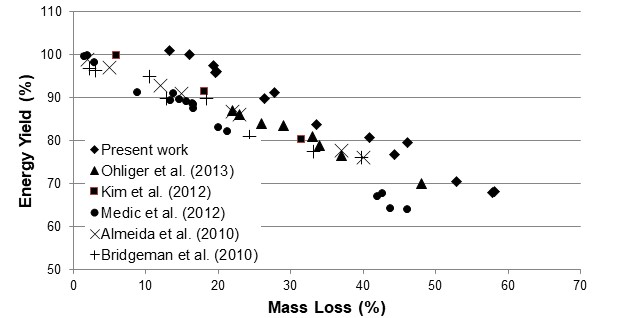Fig. 4. Energy yield versus mass loss from different publications

Proximate Analysis

Table 5 presents the proximate analyses of the torrefied samples. The final moisture content for the torrefied biomass was about 1.8%. This value is lower than the value presented by Bridgeman et al. (2010). The volatile matter shows a strong change at about 275 °C; below 275 °C the variation was about 7%, while above 275 °C it was about 20%. The ash content for the red oak used here was extremely small and was lower than that that presented by Prins et al. (2006b) using beech, willow, and straw. Carrasco et al. (2013) used a fluidized reactor with red oak and presented a maximum content of 43.8% fixed carbon for operating conditions of 330 °C and 10 min; this suggests that the fluidized reactor exhibited much faster reaction kinetics than that seen in the rotary kiln reactor.

Table 5. Proximate Analyses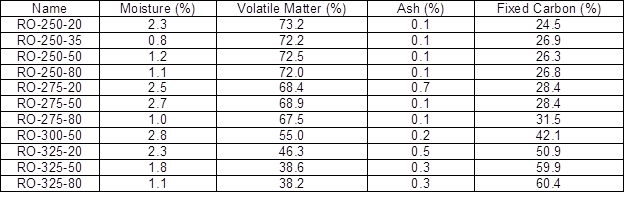Ultimate Analysis

According to Table 6, there was a difference of 0.4% between the raw material and the torrefied biomass in terms of nitrogen. Sulfur was not detected. With respect to hydrogen, the difference between the torrefied and raw red oak biomass was 2.4%. The composition of carbon and oxygen in the torrefied biomass at 325 °C and 20 min were 66.6% and 26.2%, respectively. These results are similar to those found by Carrasco et al. (2013), where they presented a composition of carbon and oxygen in the torrefied red oak of 66.1% and 26.7% at 330 °C and 10 min.

Table 6. Elemental Composition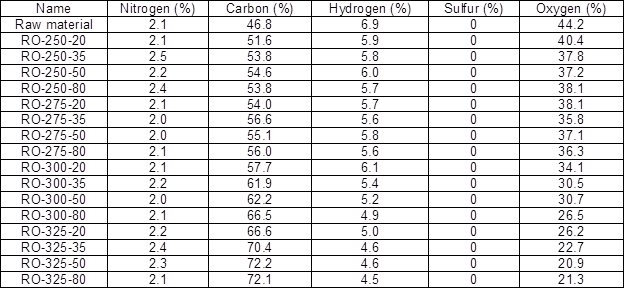In Table 7 the molar elemental ratios are presented for the red oak before and after the torrefaction process.

Table 7. Molar Ratio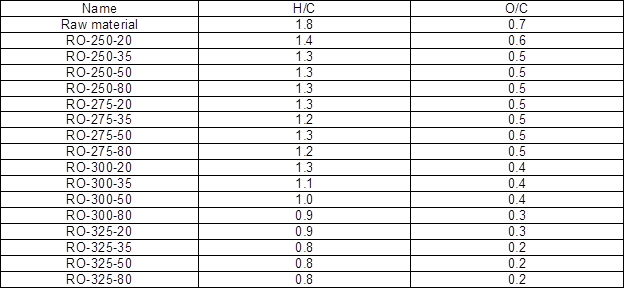H/C: molar ratio between hydrogen and carbon; O/C: molar ratio between oxygen and carbon

The results presented are consistent with those of other authors (Bergman et al. 2005; Bridgeman et al. 2010; Phanphanich and Mani 2011), although the torrefaction processes were significantly different. For instance, the experimental data shown by Carrasco et al. (2013) presented a molar ratio H/C of 1.4 and O/C of 0.6 at 230 °C and 10 min; however, in this work the same molar ratios were obtained at 250 °C and 20 min. This suggests that the torrefied materials would present similar molar ratios H:C:O, but the results depend on the particular method of torrefaction.

Kinetic Model for the Torrefaction of Red Oak

Figure 5 shows the experimental data for the total solid fraction at different temperatures (250, 275, 300, and 325 °C) as a function of residence times (20, 35, 50, and 80 min). The total solid fraction after torrefaction presents an inverse relationship with the temperature. For kinetic calculations, it is fundamental to know . More details on the calculation of a kinetic model are presented in Carrasco et al. (2013).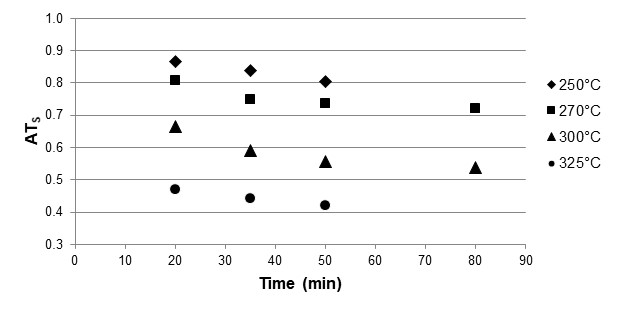Fig. 5. Total solid fraction as a function of time at different torrefaction temperatures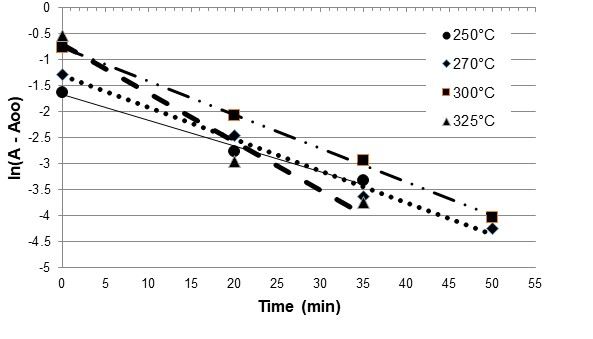Fig. 6. Logarithm of the volatile mass fraction versus time at different torrefaction temperatures

An inverse linear relationship between the logarithm of the volatile mass fraction (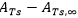) versus time at different torrefaction temperatures is observed from Figure. 6; this linear relationship allows the application of Eq. 3 to determine the reaction rate constants as a function of the torrefaction temperatures. Figure 7 was obtained using the Arrhenius equation (Eq. 4).

Figure 7 presents the plot of the natural log of different reaction rate constants as a function of torrefaction temperature. The linear correlation coefficient (r) obtained is about 0.95, which represents a strong positive linear correlation between ln(k) and 1/T.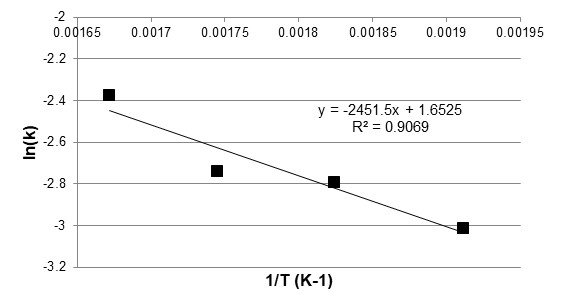Fig. 7. Parameters of the Arrhenius equation

Therefore, the kinetic model obtained for the torrefaction process of red oak (Quercus rubra) using a rotary kiln reactor is the following,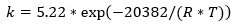, (10)

where k is the reaction rate constant (min−1), is the universal gas constant, equal to 8.314 (J mol-1K-1), and is temperature (K). The frequency factor for the torrefaction process is 5.22 min-1, and the activation energy is 20.38 kJ/mol.

It is important to analyze the error between the theoretical and empirical data; Figure 8 presents the plot of the empirical total solids fraction and the theoretical fraction of total solids obtained using Eq. 3. The maximum error presented is roughly 7.5% at a torrefaction temperature of 325 °C and a residence time of 20 min. The error is larger than that obtained by Carrasco et al. (2013), where a similar kinetic model had a maximum error of 1.3%. According to Carrasco et al.(2013), the activation energy and frequency factor vary widely, from 18 to 173.7 kJ/mole and 3.2 to 7.14×1013 min-1, respectively; these important variations are caused by differences in heating conditions, raw materials with varied characteristics, various experimental devices, particular operating conditions, and different mathematical methods to fit the laboratory results.

Using the same raw material (red oak) and the same mathematical method to fit the experimental data, the work performed here is compared with the previous work presented by Carrasco et al. (2013). The main variations between both experiments are the particle size and the equipment used to perform the torrefaction: a bench-scale fluidized reactor previously, and a pilot rotary kiln reactor in the present study.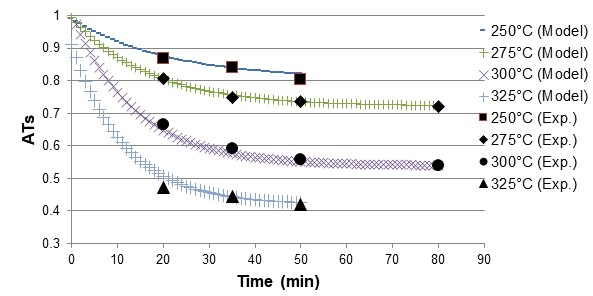Fig. 8. Fraction of total solids after torrefaction: a comparison of experimental and calculated results at torrefaction temperatures of 250, 275, 300, and 325 °C

Figure 9 presents a comparison between the apparent reaction rate constant at temperatures of 250 °C (523.15 K), 275 °C (548.15 K), 300 °C (573.15 K), and 325 °C (598.15 K) for the kinetic data published previously by Carrasco et al. (2013) and the present work. The variation of the observed activation energy and frequency factor between Carrasco et al. (2013) and this work were from 11.9 to 20.4 kJ/mol and 2.57 to 5.22 min-1, respectively. The apparent reaction rate constant (as shown in Fig. 9) determined in this study is lower than that presented by Carrasco et al. (2013) for temperatures between 250 and 325 °C; the apparent reaction rate constant decreased by about 71%. From these results it can be concluded that for the same raw material, different particle sizes, heating conditions and experimental devices may promote important changes in the heat and mass transfer characteristics, which in turn affect the apparent reaction rate constant.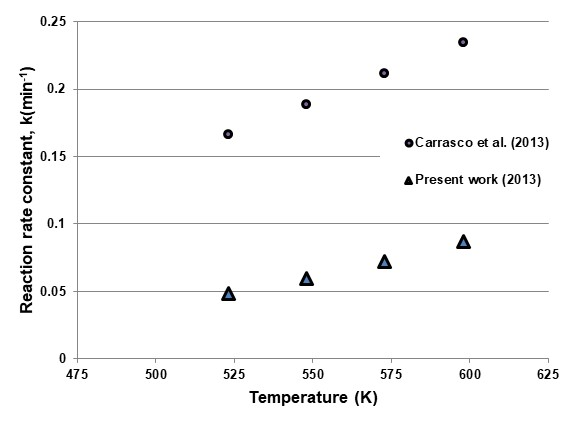Fig. 9. Comparison of reaction rate coefficients as a function of torrefaction temperature

This lower reaction rate is caused by the fact that the torrefaction process in a rotary kiln reactor presents lower mass and heat-transfer coefficients than a fluidized reactor; this is consistent with the comments presented by Li et al. (2012), who mentioned that the torrefaction of biomass in fluidized bed reactors offers improved heating efficiency.

CONCLUSIONS

1. For the torrefaction of red oak in a pilot rotary kiln reactor, a simple one-step reaction with first-order kinetics at a temperature between 250 and 325 °C was fit and showed a maximum error of roughly 7.5% at 325 °C. A similar kinetic model was used for the torrefaction of red oak in the bench-scale fluidized reactor, but the error was much less, about 1.3% at 330 °C.
2. The observed reaction rate constant, k (min-1) for the torrefaction of red oak (Quercus rubra) using a pilot rotary kiln reactor is: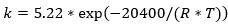,

where is the universal gas constant, 8.314 (Jmol-1K-1), and T is temperature (K). With this rate, it is possible to determine the mass loss for red oak during the torrefaction process in a pilot rotary kiln reactor at a temperature between 250 and 325 °C. Apparent kinetic parameters of a similar magnitude were obtained in a fluidized reactor; however, the reaction rate constant was 71% lower in the pilot rotary kiln reactor, indicating increased heat and mass-transfer resistances.

1. A significant incremental change of the energy content for torrefied red oak when using a rotary kiln reactor occurred between 275 and 300 °C; the energy yield changed from 97.5% to 83.6%, respectively. These conditions however depend on the heating conditions, the type of raw materials, the various experimental devices, and the specific operating conditions.
2. The molar ratios H:C:O for torrefied red oak presented a behavior independent of the experimental reactors for the temperature range between 250 and 325 °C. An inverse relationship was found between energy yield and mass loss: when the mass loss increased from 19.3% to 58.1%, the energy yield decreased from 97.5% to 68.3%.
3. For the torrefaction of red oak, the bench-scale fluidized reactor presented higher mass loss than the rotary kiln reactor; for the fluidized reactor at 330 °C and 30 min, the mass loss was 75.8%, and for the rotary kiln reactor at 325 °C and 80 min, the mass loss was 57.9%, again indicating the significant influence of the particle size and the reactor configuration.

ACKNOWLEDGEMENTS

This research was funded by a grant from NIFA-McStennis WVA00098 and the WVU-Energy Research Grant (ERG) Program; Award NT10042R- Project 10013855.

REFERENCES CITED

Alankangas, E. (2013). “Sector – production of solid sustainable energy carriers from biomass by means of torrefaction,” R&D, http://www.vtt.fi/files/projects/biohiili/eija_alakangas.pdf‎. (accessed Aug 14, 2013).

Almeida, G., Brito, J. O., and Perré, P. (2010). “Alterations in energy properties of eucalyptus wood and bark subjected to torrefaction: The potential of mass loss as a synthetic indicator,” Bioresource Technol. 101(24), 9778-9784.

Arias, B., Pevida, C., Fermoso, J., Plaza, M. G., Rubiera, F., and Pis, J. J. (2008). “Influence of torrefaction on the grindability and reactivity of woody biomass,” Fuel Process Technol. 89(2), 169-175.

ASTM D2015. (2000). Standard Test Method for Gross Calorific Value of Coal and Coke by the Adiabatic Bomb Calorimeter.

ASTM D3172-07. (2007). Standard Practice for Proximate Analysis of Coal and Coke.

ASTM D3176. (1989). Standard Practice for Ultimate Analysis of Coal and Coke.

Aziz, M. A., Sabil, K. M., Uemura, Y., and Ismail, L. (2012). “A study on torrefaction of oil palm biomass,” J. Appl. Sci. 12(11), 1130-1135.

Barooah, J. N., and Long, V. D. (1976). “Rates of thermal decomposition of soma carbonaceous materials in a fluidized bed,” Fuel 55(2), 116-120.

Basu, P., Rao, S., and Dhungana, A. (2013). “An investigation into the effect of biomass particle size on its torrefaction,” The Canadian Journal of Chemical Engineering 91(3), 466-474.

Bates, R. B. (2012). “Modeling the coupled effects of heat transfer, thermochemistry, and kinetics during biomass torrefaction,” MS thesis, Massachusetts Institute of Technology, available from: http://dspace.mit.edu/handle/1721.1/70433 (accessed May 21, 2014).

Bates, R. B., and Ghoniem, A. F. (2012). “Biomass torrefaction: Modeling of volatile and solid product evolution kinetics,” Bioresource Technol. 124(11), 460-469.

Bates, R. B., and Ghoniem, A. F. (2013). “Biomass torrefaction: Modeling of reaction thermochemistry,” Bioresource Technol. 134(4), 331-340.

Bergman, P. C. A., and Kiel, J. H. A. (2005). “Torrefaction for biomass upgrading. Energy Research Centre of the Netherlands (ECN),” Publication No. ECN-RX-05-180, available from: http://www.ecn.nl/docs/library/report/2005/rx05180.pdf. (accessed Aug 14, 2013).

Bergman, P. C. A., Boersma, A. R., Kiel, J. H. A., Prins, M. J., Ptasinski, K. J., and Janssen, F. J. J. G. (2005). “Torrefaction for entrained-flow gasification of biomass,” Report ECN-C-05-067, The Netherlands. P.50, available from: ftp://nrg-nl.com/pub/www/library/report /2005/c05067.pdf. (accessed Aug 14, 2013).

Bridgeman, T. G., Jones, J. M., Shield, I., and Williams, P. T. (2008). “Torrefaction of reed canary grass, wheat straw and willow to enhance solid fuel qualities and combustion properties,” Fuel 87(6), 844-856.

Bridgeman, T. G., Jones, J. M., Williams, A., and Waldron, D. J. (2010). “An investigation of the grindability of two torrefied energy crops,” Fuel 89(12), 3911-3918.

Carrasco, J. C., Oporto, G. S., Zondlo, J., and Wang, J. (2013). “Torrefaction kinetics of red oak (Quercus rubra) in a fluidized reactor,” BioResources 8(4), 5067-5082.

Chen, W. H., and Kuo, P. C. (2011). “Isothermal torrefaction kinetics of hemicellulose, cellulose, lignin and xylan using thermogravimetric analysis,” Energy36(11), 6451-6460.

Ciolkosz, D., and Wallace, R. (2011). “A review of torrefaction for bioenergy feedstock production,” Biofuel. Bioprod. Bior. 5(3), 317-329.

Cuellar, A. D. (2012). “Plant power – The cost of using biomass for power generation and potential for decreased greenhouse gas emissions,” MS thesis, Massachusetts Institute of Technology, available from: http://sequestration.mit.edu/pdf/Amanda Cuellar_Thesis_June 2012.pdf‎. (accessed Aug 14, 2013).

Deng, J., Wang, G. J., Kuang, J. H., Zhang, Y. L., and Luo, Y. H. (2009). “Pretreatment of agricultural residues for co-gasification via torrefaction,” J. Anal. Appl. Pyrol. 86(2), 331-337.

Di Blasi, C., and Branca, C. (2001). “Kinetics of primary product formation from wood pyrolysis,” Ind. Eng. Chem. Res. 40(23), 5547-5556.

Fisher, E. M., Dupont, C., Darvell, L. I., Commandre, J. M., Saddawi, A., Jones, J. M., Grateau, M., Nocquet, T., and Salvador, S. (2012). “Combustion and gasification characteristics of chars from raw and torrefied biomass,” Bioresource Technol. 119(9), 157-165.

Kiel, J. H. A. (2013). “Torrefaction – product quality optimization in view of logistics and end-use,” ECN –L -13 -028, available from: http://www.ecn.nl/docs/library/report/ 2013/l13028.pdf‎. (accessed Aug 14, 2013).

Kim, Y. H., Lee, S. M., Lee, H. W., and Lee, J. W. (2012). “Physical and chemical characteristics of products from the torrefaction of yellow poplar (Liriodendron tulipifera),” Bioresource Technol. 116(6), 120-125.

Kleinschmidt, C. (2011). “Overview of international developments in torrefaction,” Central European Biomass Conference, Graz, Austria, available from: http://www.bioenergytrade.org /pastevents/graz.html. (accessed Aug 14, 2013).

Levine, E. (2011). “Biomass densification for cofiring applications,” Meeting Presentations from the March 2–3, 2011, Biomass Research and Development Technical Advisory Committee Meeting, available from: http://biomassboard.gov/biomass/brdi/pdfs/levine_btac_ 3-3-11.pdf. (accessed Aug 14, 2013).

Li, H., Liu, X. H., Legros, R., Bi, X. T. T., Lim, C. J., and Sokhansanj, S. (2012). “Torrefaction of sawdust in a fluidized bed reactor,” Bioresource Technol.103(1), 453-458.

Medic, D., Darr, M., Shah, A., Potter, B., and Zimmerman, J. (2012). “Effects of torrefaction process parameters on biomass feedstock,” Fuel 91(1), 147-154.

Ohliger, A., Forster, M., and Kneer, R. (2013). “Torrefaction of beechwood: A parametric study including heat of reaction and grindability,” Fuel 104(1), 607-613.

Oliveira, T., and Rousset, P. L. A. (2009). “Effects of torrefaction on energy properties of eucalyptus grandis wood,” Cerne 15(4), 446-452.

Pyle, D. L., and Zaror, C. A. (1984). “Heat transfer and kinetics in the low temperature pyrolysis of solids,” Chemical Engineering Science 39, 147-158.

Peng, J., Lim, J., Bi, X. T., and Sokhansanj, S. (2012). “Development of torrefaction kinetics for British Columbia softwoods,” Int. J. Chem. Reactor Eng. 10(1), 1-37.

Phanphanich, M. and Mani, S. (2011). “Impact of torrefaction on the grindability and fuel characteristics of forest biomass,” Bioresource Technol. 102(2), 1246-1253.

Prins, M., Ptasinski, K. J., and Janssen, F. J. J. G. (2006a). “Torrefaction of wood Part 1: Weight loss kinetics,” J. Anal. Appl. Pyrol. 77(1), 28-34.

Prins, M., Ptasinski, K. J., and Janssen, F. J. J. G. (2006b). “Torrefaction of wood Part 2: Analysis of products,” J. Anal. Appl. Pyrol. 77(1), 35-40.

Reina, J., Velo, E., and Puigjaner, L. (1998). “Kinetic study of the pyrolysis of waste wood,” Ind. Eng. Chem. Res. 37(11), 4290-4295.

Ren, S., Lei, H., Wang, L., Bu, Q., Chen, S., and Wu, J. (2013). “Thermal behaviour and kinetic study for woody biomass torrefaction and torrefied biomass pyrolysis by TGA,” Biosyst. Eng. 116(4), 420-426.

Samuelsson, L. N., Engvall, K., and Moriana, R. (2013). “A methodology to model the kinetics of torrefaction,” KTH Royal Institute of Technology, Stockholm, Sweden, available from: http://www.gastechnology.org/tcbiomass2013/tcb2013/13-Samuelsson-tcbiomass2013-presentation-Fri.pdf. (accessed Aug 14, 2013).

TenWolde, A., McNatt, J. D., and Krahn, L. (1988). “Thermal properties of wood and wood panel products for use in buildings,” DOE/USDA-21697/1/ORNL/ Sub/87-21697/1, available form: http://web.ornl.gov/info/reports/1988/3445602 823407.pdf (accessed May 21, 2014).

Thurner, I., and Mann, U. (1981). “Kinetic investigation of wood pyrolysis,” Ind. Eng. Chem. Process. Des. Dev. 20(3), 482-488.

Tiffany, D. G. (2013). “Economics of torrefaction plants and businesses buying their products,” University of Minnesota, available from: http:// heating themidwest.org/wp-content/uploads//Tiffany.pdf. (accessed Aug 14, 2013).

Zheng, A., Zhao, Z., Chang, S., Huang, Z., He, F., and Li, H. (2012). “Effect of torrefaction temperature on product distribution from two-staged pyrolysis of biomass,” Energ. Fuel 26(5), 2968-2974.

Article submitted: March 14, 2014; Peer review completed: May 1, 2014; Revised version received: July 8, 2014; Accepted: July 10, 2014; Published: July 21, 2014.

APPENDIX A: Calculations of Biot and Pyrolysis Numbers

A.1) Properties of red oak

Density of red oak: 660 [kg/m3] (TenWolde et al. 1988).

Specific heat of wood: 1,670 [J/kg K] (Pyle and Zaror 1984).

Conductivity of red oak (oven dry): 0.15 [W/m K] (TenWolde et al. 1988).

A.2) Particle characteristic length/ red oak specific information

Using the biggest particle with diameter (9.51 mm) and length (100 mm) as cylinder, the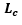is equal to 2.27×10-3 [m]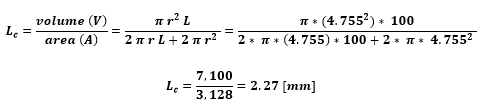A.3) External heat transfer coefficient

We may calculate h with experimental data by means of a lumped analysis since Bi <1. The temperature of the samples inside the reactor (T) increased from ambient temperature (15°C) to 200°C in approximately 25 minutes. Considering the temperature inside the furnace (Tf) is equal to 250°C, the energy balance of one particle of red oak is: (same characteristics as in the previous section)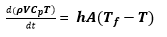Eq (A.3.1)

Boundary conditions: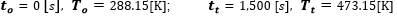Considering constant the parameters: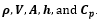Integrating of Eq (A.3.1).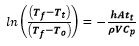Eq (A.3.2)

Reordering the Eq (A.3.2)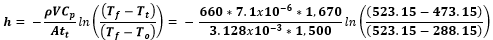Then, the heat transfer coefficient obtained is equal to 2.58 [W/m2 K]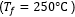.

According Pyle and Zaror (1984) the value of h presented in their work lies between 8.4 and 100 [W/m2 K]. Therefore, for our evaluation we used the value of 8.4 [W/m2 K]. It should also be noted that this value of h is quite small indicating a large thermal resistance outside the particle.

A.4) Reaction rate constant

According Bates (2012)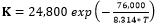[1/s], then evaluating to 250 °C: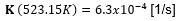[1/s].

According to the results of this publication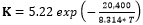[1/min], then evaluation at 250, 275, 300, and 325 °C: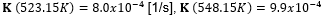[1/s],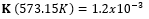[1/s], and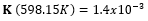[1/s]. We may use our results.

A.5) Biot Number

Considering the information presented above, we can evaluate the Biot Number.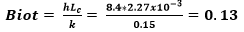A.6) Py, and Py’ Numbers at 250, 275, 300, and 325 °C

Considering the information presented from A.1 to A.4, it is possible to evaluate the Pyrolysis Number at different torrefaction temperatures, using Eqs. (2) and (3).

Table 8. Py, and Py’ Numbers at 250, 275, 300, and 325 °C